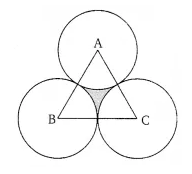# The area of an equilateral triangle ABC is 17320.5 cm2. With each vertex of the triangle

The area of an equilateral triangle ABC is 17320.5 cm2. With each vertex of the triangle as centre, a circle is drawn with radius equal to half the length of the side of the triangle (see figure). Find the area of the shaded region.
(Use π = 3.14 and √ 3 = 1.73205).